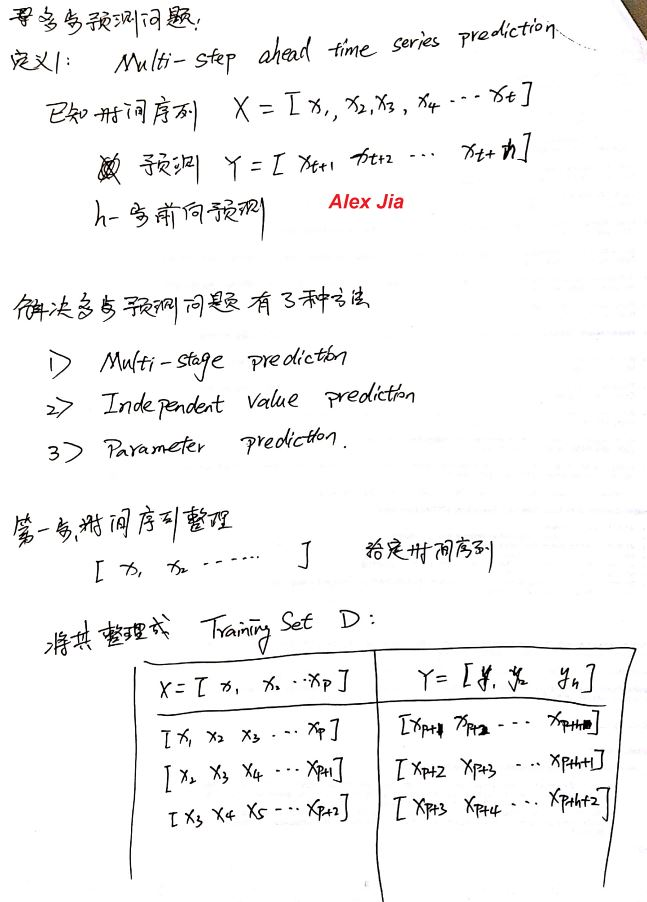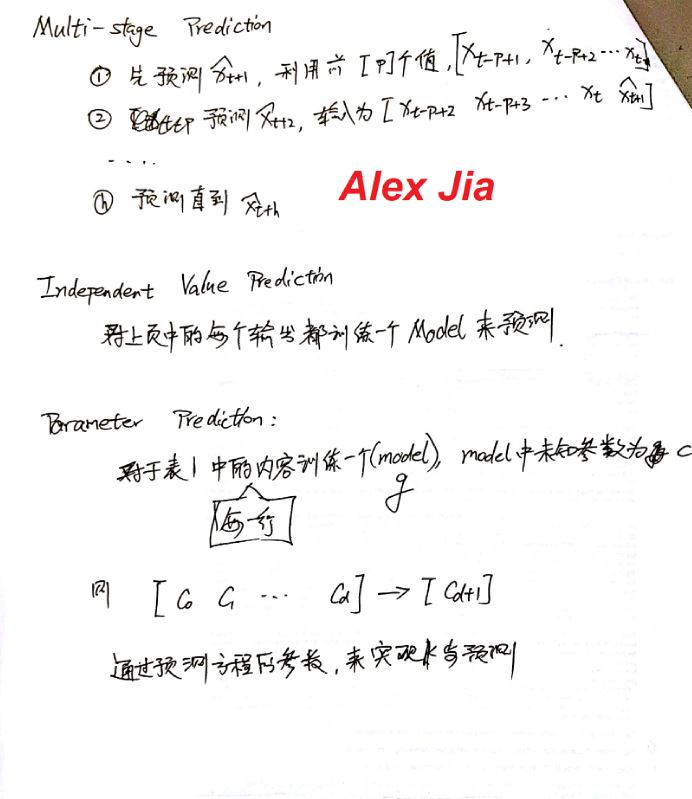• 的直接多步预测模型; 给出了易于实现的动量梯度学习算法, 并对其收敛性进行了验证. 仿真结果表明, 直接多步预 测不依赖于单步预测的结果, 对比单步预测模型能快速、准确地预测船舶横摇运动时间序列, 具有更好的...
• Direct Multi-step Forecast Strategy. 同时建多个模型，缺点：没有考虑预测的相邻两个值的相关性 Recursive Multi-step Forecast Strategy.缺点：预测误差往后迅速积累，数据...
https://machinelearningmastery.com/multi-step-time-series-forecasting/

Direct Multi-step Forecast Strategy.   同时建多个模型，缺点：没有考虑预测的相邻两个值的相关性
Recursive Multi-step Forecast Strategy.缺点：预测误差往后迅速积累，数据会很快没有意义
Direct-Recursive Hybrid Multi-step Forecast Strategies.前两者结合起来做预测，

Multiple Output Forecast Strategy.一个模型，一次预测多个输出

转载于:https://www.cnblogs.com/eagou/p/9307766.html
展开全文• 在对SCATS线圈数据进行预处理的基础上,设计了一种基于动态神经网络的短时多步预测双层模型,包括基于NARX(Nonlinearautoregressivemodelwithexogenousinputs)神经网络的多步预测方法以及基于FTD(Focusedtime-...
• 提出了基于多步预测控制方法的多变量非线性神经网络逆控制方案。利用预测模型对系统动态特性进行预测，使用一个带有时延因子的前馈神经网络作为控制器，利用多步预测性能指标对其在线训练，实现神经网络逆系统；在...神经网络 非线性系统
• 针对传感网环境监测应用采集的时间序列数据，提出了一种新的基于高斯过程模型的多步预测方法，实现了对未来时刻的环境监测数据的预测。高斯过程模型通过核函数描述数据的特性，通过对环境监测数据的经验模态分解，...时间序列 高斯过程
• support vectormachine，LS—SVM)的话务量预测方法，采用自相关分析法确定LS—SVM建模输入样本的嵌入维数和延迟时间，最 大限度地保留历史信息并降低样本的维数；在此基础上，以最少量预测值代替真实值构成多步预测...
• 针对较强非线性的控制问题, 提出一种以RBF 神经网络为模型的多步预测控制方法. 构建多步预测模型, 并给出预测误差关于控制序列的雅可比矩阵的计算方法. 利用Levenberg-Marquardt(L-M) 算法设计滚动优化策略, 过误差...
• 原文链接：原文 There are at least four commonly used strategies for making multi-step forecasts. They are: 1.Direct Multi-step Forecast Strategy. The direct method involves developing a separate model...
原文链接：原文
There are at least four commonly used strategies for making multi-step forecasts.
They are:
1.Direct Multi-step Forecast Strategy.
The direct method involves developing a separate model for each forecast time step.
In the case of predicting the temperature for the next two days, we would develop a model for predicting the temperature on day 1 and a separate model for predicting the temperature on day 2.
prediction(t+1) = model1(obs(t-1), obs(t-2), ..., obs(t-n))
prediction(t+2) = model2(obs(t-2), obs(t-3), ..., obs(t-n))

2.Recursive Multi-step Forecast Strategy.
The recursive strategy involves using a one-step model multiple times where the prediction for the prior time step is used as an input for making a prediction on the following time step.
In the case of predicting the temperature for the next two days, we would develop a one-step forecasting model. This model would then be used to predict day 1, then this prediction would be used as an observation input in order to predict day 2.
prediction(t+1) = model(obs(t-1), obs(t-2), ..., obs(t-n))
prediction(t+2) = model(prediction(t+1), obs(t-1), ..., obs(t-n))

Because predictions are used in place of observations, the recursive strategy allows prediction errors to accumulate such that performance can quickly degrade as the prediction time horizon increases.
3.Direct-Recursive Hybrid Multi-step Forecast Strategies.
The direct and recursive strategies can be combined to offer the benefits of both methods.
For example, a separate model can be constructed for each time step to be predicted, but each model may use the predictions made by models at prior time steps as input values.
We can see how this might work for predicting the temperature for the next two days, where two models are used, but the output from the first model is used as an input for the second model.
prediction(t+1) = model1(obs(t-1), obs(t-2), ..., obs(t-n))
prediction(t+2) = model2(prediction(t+1), obs(t-1), ..., obs(t-n))

Combining the recursive and direct strategies can help to overcome the limitations of each.
4.Multiple Output Forecast Strategy.
The multiple output strategy involves developing one model that is capable of predicting the entire forecast sequence in a one-shot manner.
In the case of predicting the temperature for the next two days, we would develop one model and use it to predict the next two days as one operation.
prediction(t+1), prediction(t+2) = model(obs(t-1), obs(t-2), ..., obs(t-n))

Multiple output models are more complex as they can learn the dependence structure between inputs and outputs as well as between outputs.
Being more complex may mean that they are slower to train and require more data to avoid overfitting the problem.


展开全文• 为了提高风电场短期风速预测的精确度以及预测尺度,提出了一种将小波分解法、经验模式分解法及最小二乘支持向量机相结合对风速时间序列进行短期多步预测建模的方法。该方法采用小波分解法对风速信号进行分解,使之分解...
• 各位前辈好。目前我想使用深度神经网络实现时间序列的多步预测，可是预测步数多了会 出现累积误差。所以有没有一种方法可以实现网络的自学习能力，使用后续的数据对网络进行再次训练。人工智能 数据挖掘 深度学习 神经网络 自然语言处理
• 递归预测值上展开, 实现对非线性系统多步预测输出值的二次逼近, 减少了预测误差. 进而通过对P ID 型多步预测 性能指标函数极小化求取控制量. 控制器与广义预测控制器结构相似, 其参数通过神经网络在线辨识获得....
• 将支持向量机技术应用于水泵的时间序列多步预测中。为提高预测精度,提出利用多个测量通道获得的多时间序列进行预测,并通过水泵电机上获得的实际测量数据进行对比分析,证明该方法在设备长期预测中具有较好的预测精度...
• 针对离散非线性系统,利用非线性激励函数的局部线性表示,提出一种可用于非线性过程的神经网络多步预测控制方法,并给出了控制律的收效性分析。该方法将非线性系统处理成简单的线性和非线性两部分,对复杂的非线性多步...
• 针对离散非线性系统, 利用非线性激励函数的局部线性表示, ...线性多步预测方程给出了直观而有效的线性形式, 并用线性预测控制方法求得控制律, 避免了复杂的非线性优化求 解. 仿真结果表明了该算法的有效性.</p>
• 利用相空间重构技术，并借助G-...计算了宁陵地区地下水位时间序列的关联维数、时间延迟和最大Lyapunov指数，将局域加权一阶多步预测模型应用于地下水位预测。预测表明，此模型可有效应用于地下水位时间序列的多步预测
• 针对混沌时间序列的多步预测, 提出了基于最大互信息(MMI) 的建模方法. 首先建立时间延迟、嵌入维 数和预测步长在相空间的最大信息量模型; 然后利用遗传算法求解并确定混沌时间序列的最佳预测结构; 最后对 ...
• 根据跳频频率序列具有混沌特性，在相空间重构理论基础上提出一种用于跳频频率序列预测的贝叶斯网络模型。该模型将重构后的整个相空间作为先验...仿真结果表明该方法具有良好的多步预测能力，并能有效地克服过拟合现象。贝叶斯网络 相空间重构
•展开全文Prediction regression
• 为了进一步改善悉尼自适应交通控制系统（Sydney coordinated adaptive traffic system，SCATS）线圈数据短时多步预测的效果，在对SCATS线圈数据进行预处理的基础上，将当前与之前若干时间间隔的交通数据及对应的时间...
• 首先应用模糊聚类方法将数据...最后将该模型用于上证股票综合指数和深证股票成分指数的多步预测和涨跌趋势预测。与典型模糊时间序列模型比较，涨跌趋势预测准确率有较大提高，多步预测结果表明模型具有较好的泛化能力。
• 针对一类P ID 型模糊控制器, 提出基于多步预测性能指标的模糊控制器参数优化设计方法. 通过最小化多 步预测性能指标和调整比例因子, 定量给出了控制器的设计准则, 对于现场操作人员具有一定的指导意义. 仿真...
• 设计一种次序优化机制,提出一类饱和受限不确定系统的次序优化多步预测控制方法.将系统的输入分解成多个子集合,在各采样周期仅对其中一个集合的输入进行优化,待优化的输入保持上一优化值.针对每个输入集合,确定闭环...
• 对网络流量时间序列进行相空间重构之后，利用一种改进的回声状态网络进行网络流量的多步预测。提出一种改进的和声搜索优化算法对回声状态网络的相关参数进行优化以提高预测精度。利用网络流量的公共数据集以及实际...网络流量 回声状态网络
• 仿真实验说明这种多步预测方法是可行的，在一定的预测精度下可同时实现对未来多个频点的有效预测。多步预测可更好地满足预测的实时性，对近年来提出的跳频通信预测干扰具有更好的工程应用价值。混沌理论
• #先上代码后补笔记# ...由亚当斯-巴什福特(Adams-Bashforth)显式预测公式和亚当斯-莫顿(Adams-Moulton)隐式修正公式组成的预测-修正(PECE)对。 function [ YMat ] = Adams( func, tvec, y_init, order ) ...

#先上代码后补笔记#
#可以直接复制粘贴调用的MATLAB函数代码！#
1. 亚当斯(Adams)预测-修正算法
由亚当斯-巴什福特(Adams-Bashforth)显式预测公式和亚当斯-莫顿(Adams-Moulton)隐式修正公式组成的预测-修正(PECE)对。

function [ YMat ] = Adams( func, tvec, y_init, order )
%　　Adams预测-修正算法，用于求解常微分初值问题
%   输入四个参数：函数句柄func（接收列向量、返回列向量），积分时间列向量tvec，初值行向量y_init，阶数order；
%   输出一个参数：数值解，每一行对应积分时间列向量的一行，各列为变量一个分量。
switch order
case '4'
row = size(tvec, 1); col = size(y_init, 2);
YMat = zeros(row, col);
YMat(1:4, :) = Runge_Kutta(func, tvec(1:4), y_init, '4');
for i=4:row - 1
stepsize = tvec(i + 1) - tvec(i);
ydiff0 = func(tvec(i), YMat(i, :).');
ydiff1 = func(tvec(i - 1), YMat(i - 1, :).');
ydiff2 = func(tvec(i - 2), YMat(i - 2, :).');
ydiff3 = func(tvec(i - 3), YMat(i - 3, :).');
y_predict = YMat(i, :).' + (55*ydiff0 - 59*ydiff1 + 37*ydiff2 - 9*ydiff3)*stepsize/24;
y_corrector = YMat(i, :).' + (9*func(tvec(i + 1), y_predict) + 19*ydiff0 - 5*ydiff1 + ydiff2)*stepsize/24;
YMat(i + 1, :) = y_corrector.';
end
end
end

转载于:https://www.cnblogs.com/gentle-min-601/p/9638186.html
展开全文• //TODO 有空了我找个例子使用python和MLP实现对比一下四种策略 参考：  4 Strategies for Multi-Step Time Series Forecasting, 2017 Machine Learning Strategies for Time Series Forecasting, 2013 ...python 机器学习
• 基于回声状态网络的混沌时间序列多步预测，宋勇 ，李贻斌，针对回声状态网络在权值学习过程中存在的病态问题，提出了一种基于正则化方法的回声状态网络学习算法。在标准误差项基础上增加一
• 为了减小车用汽油机空燃比传输延迟对空燃比控制精度的影响，提出一种基于Elman神经网络的空燃比多步预测模型。通过对空燃比数学模型的分析，确定神经网络空燃比多步预测模型的输入向量，同时，为了提高过渡工况空燃...
• 提出了一种基于2次多项式核函数支持向量机的多步预测控制方法。通过黑箱辨识和线性化技术得到非线性系统的近似模型，根据预测控制机理，最小化滚动时域的二次型目标函数，利用模型算法控制的方法得到控制器的解析...
• 提出的多步预测控制方法，具有很好的全局识别特点和较高的推广预测能力．工程实例分析表明，IPSO-Elamn算法在桩筏(箱)基础沉降的非线性系统动态辨识和在线预测应用方面，具有良好的预测精度，满足工程实际需要．